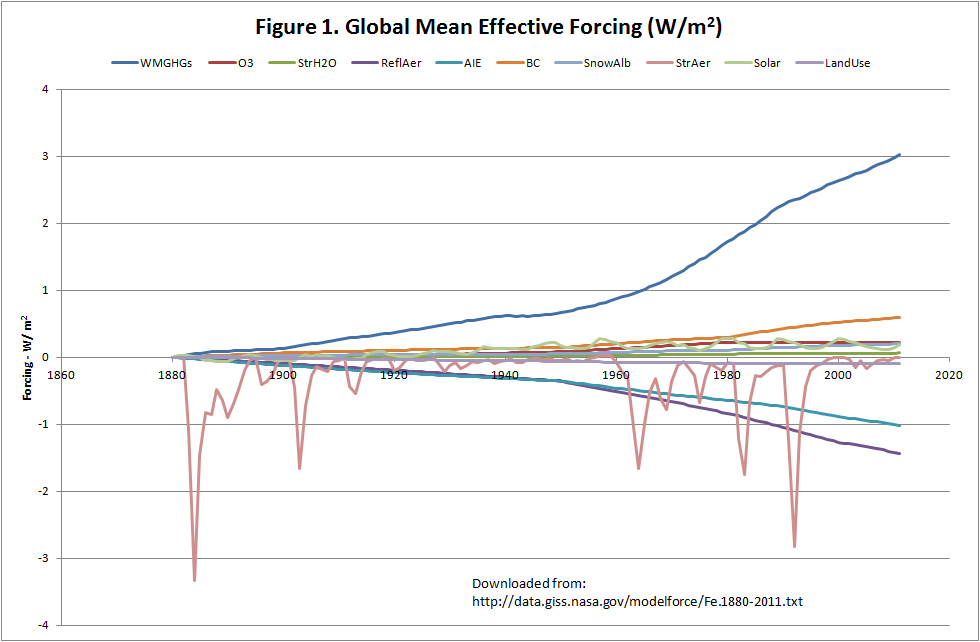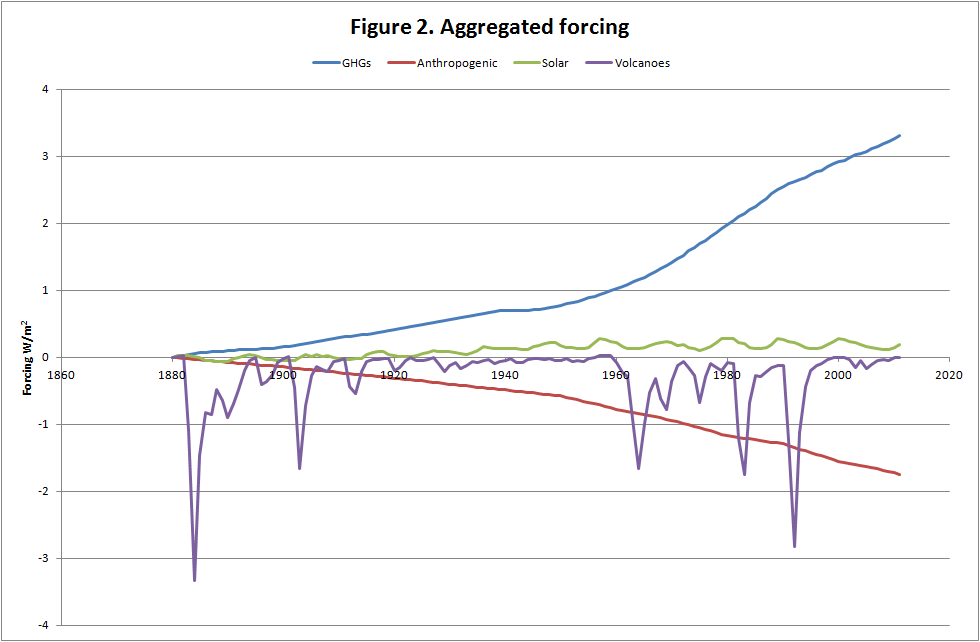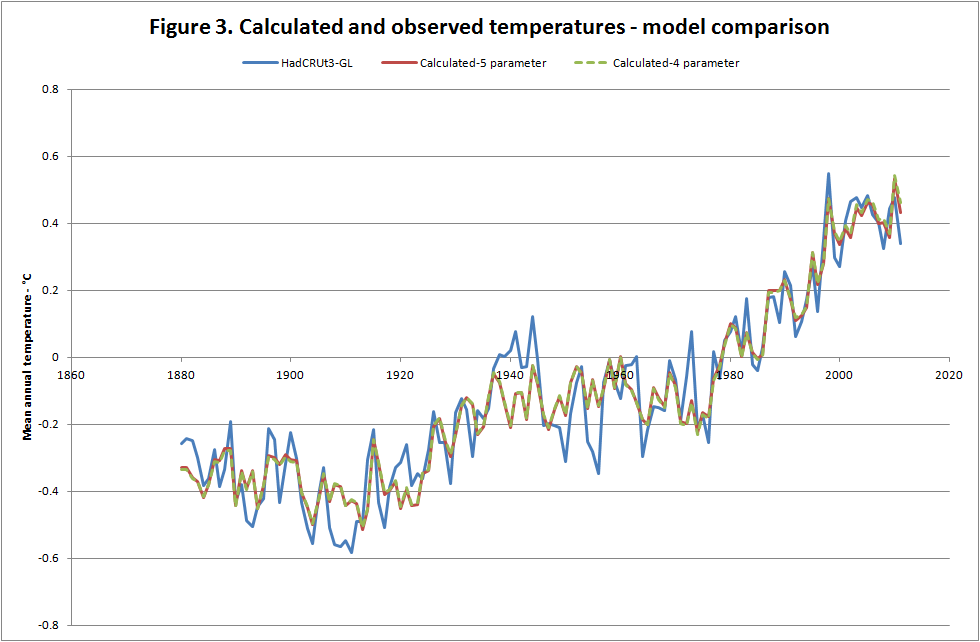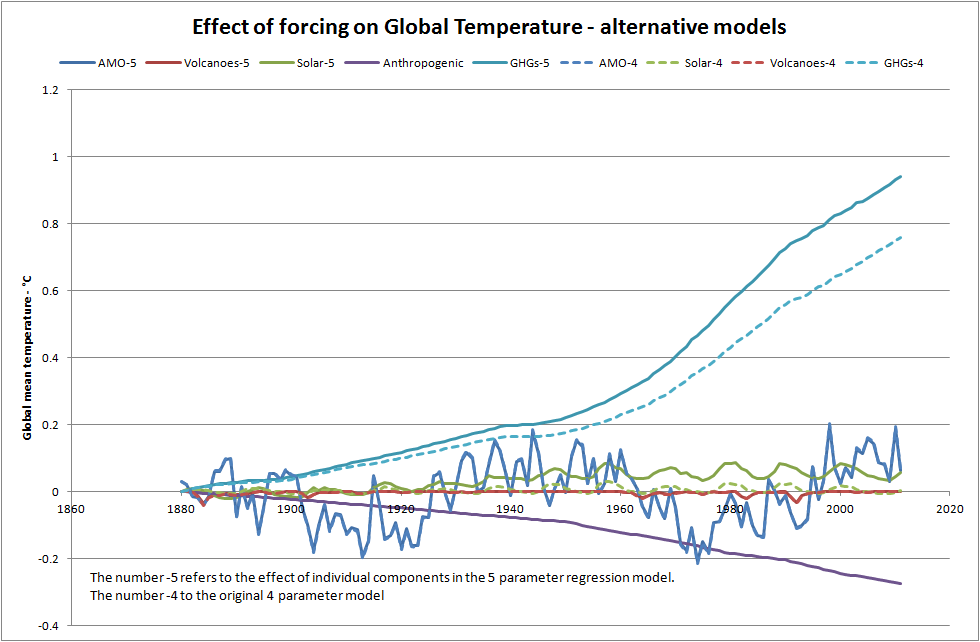Thursday, November 15, 2012

AMO AND ANTHROPOGENIC AEROSOLS

It has been pointed out that the model I described in my earlier post (Climate and the Atlantic Multidecadal Oscillation) ignored anthropogenic aerosols. Here I look at the effect of adding these into the model.

Data
The data used were downloaded from http://data.giss.nasa.gov/modelforce/Fe.1880-2011.txt. They were used in J. Hansen, et al. (2007) "Climate simulations for 1880-2003 with GISS model E", Clim Dyn, 29: 661-669 and J. Hansen, et al. (2011) "Earth's energy imbalance and implications, Atmos Chem Phys, 11, 13421-13449.

Figure 1 shows the individual components.The individual components are:

WMGHGs – Well mixed greenhouse gases
O3 - Ozone
StrH2O – Stratospheric H­2O
ReflAer – Reflective Aerosols
AIE – Aerosol indirect effect
BC – Black carbon
SnowAlb – Snow albedo
StrAer – Stratospheric Aerosols (Volcanoes)
LandUse – Land Use.

To run a regression model with these 10 parameters plus the Atlantic Multidecadal Oscillation (AMO) would be nonsense. This would be particularly so since many of the components are highly correlated; the regression coefficient between WMGHGs and AIE is 0.98 for example. So I aggregated them into 4 groups. The first three (WMGHGs, O3 and StrH2O) I grouped as GHGs. Stratospheric aerosols and solar were treated separately. This gave 3 parameters which had exact equivalent in the original 4-parameter model. The fourth parameter was the sum of all the other components. The aggregated parameters are shown on Figure 2.The fifth and final parameter was the AMO.

For temperature I used the HadCRUT3 global data set. I am aware that this has been superseded by the version 4 but for consistency with the earlier posting I am sticking to it.

Results
The accuracy of the two models was almost identical. This can be seen on figure 3.In terms of accuracy, the 4-parameter model (for the period 1880 to 2011) explained 89.3% of the variance and the 5-parameter model explained 89.5%, confirming the similar accuracy of the two models.

Components
The comparison of observed and calculated temperatures does not describe how the values were actually calculated. Figure 4 shows the effect of each component on temperature.In the above the use of ‘-5’ refers to 5 parameter model and ‘-4’ to the original 4 parameter model. The main differences between the models are:

• The effect of GHGs is larger in the 5-parameter model, the difference being largely due to the effect of the anthropogenic factors.
• Solar effects are slightly higher in the 5-parameter models.
• The effect of volcanoes is minimal in both models.
• The influence of the AMO is virtually identical in both models.

The coefficients for the 5 independent variables were:

Since the all parameters, except for AMO, are expressed as W/m2 and if the forcing sensitivity is close to the accepted value of 3.2 W/m2/°C then each coefficient should have the value 0.3125 °C/W/m2 (that is 1/3.2). In this case the parameters for GHGs and solar irradiance are close to that value. That for volcanoes is much lower than expected. The parameter for combined anthropogenic effects is also lower than expected but has wide error bands so the ‘expected’ figure is within the 95% range.

Considering the critical period from 1976 to 2005, which had the largest increase, the observed temperature increases were as given in the table below.

Considering the period of rapid temperature rise, the original 4-parameter model suggested that 49% of the rise was due to GHGs, the equivalent figure for the 5-parameter model is 59%.

These findings are consistent with those reported in Zhou, J., and K. Tung, 2012: Deducing Multi-decadal Anthropogenic Global Warming Trends Using Multiple Regression Analysis. J. Atmos. Sci. doi:10.1175/JAS-D-12-0208.1, in press. I will discuss this paper in another posting.

Conclusions
The conclusion remains as before. It is not clear whether the AMO is the ‘heart’ of the system and is driving global temperature or whether it is the ‘pulse’ and is an indicator another driver. What is clear that global temperatures reflect changes in the AMO but the AOGCMs used by the IPCC do not consider the AMO. In particular the AOGCMs simulated reasonably accurately the large temperature increase from 1976 to 2005 but attribute all the increase to GHGs. As a consequence, as pointed out in this and previous postings, and some recent peer-reviewed papers, temperature increases in the coming decades are likely to be lower than the IPCC projections.